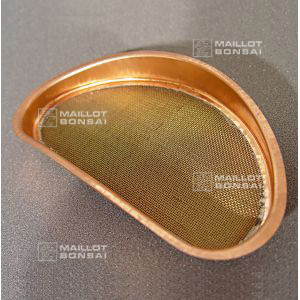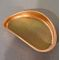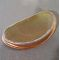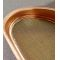Start your cart here complete your order on Maillot-Erable or Japon-Import and reduce your shipping costs##### The Japanese Bonsai specialist
Direct order Contact Help / Services Newsletter# Filter for 4-litre watering can 136*72 mm

› Bonsai tools › Watering equipment › Copper watering canref. : 658132,00

Available quantity : 1Order

###### Description

Length: 136 mm. Width: 72 mm. Copper thread grid to fit our 4-litre Japanese watering can and filter out impurities.

#watering 5.8 #copper 3.6 #filter 3.6 #litre 3.5 #impurities 2 #japanese 1.8 #length 1.6 #thread 1.6 #width 1.5 #grid 1.4

Formule
(( ROUND((CHAR_LENGTH(b.article_nom)-CHAR_LENGTH(REPLACE(b.article_nom, 'watering', '')))/LENGTH('watering')) + ROUND((CHAR_LENGTH(b.article_description)-CHAR_LENGTH(REPLACE(b.article_description, 'watering', '')))/LENGTH('watering')) ) * 3.8) + (( ROUND((CHAR_LENGTH(b.article_nom)-CHAR_LENGTH(REPLACE(b.article_nom, 'filter', '')))/LENGTH('filter')) + ROUND((CHAR_LENGTH(b.article_description)-CHAR_LENGTH(REPLACE(b.article_description, 'filter', '')))/LENGTH('filter')) ) * 3.6) + (( ROUND((CHAR_LENGTH(b.article_nom)-CHAR_LENGTH(REPLACE(b.article_nom, 'litre', '')))/LENGTH('litre')) + ROUND((CHAR_LENGTH(b.article_description)-CHAR_LENGTH(REPLACE(b.article_description, 'litre', '')))/LENGTH('litre')) ) * 3.5) + (( ROUND((CHAR_LENGTH(b.article_nom)-CHAR_LENGTH(REPLACE(b.article_nom, 'impurities', '')))/LENGTH('impurities')) + ROUND((CHAR_LENGTH(b.article_description)-CHAR_LENGTH(REPLACE(b.article_description, 'impurities', '')))/LENGTH('impurities')) ) * 2) + (( ROUND((CHAR_LENGTH(b.article_nom)-CHAR_LENGTH(REPLACE(b.article_nom, 'japanese', '')))/LENGTH('japanese')) + ROUND((CHAR_LENGTH(b.article_description)-CHAR_LENGTH(REPLACE(b.article_description, 'japanese', '')))/LENGTH('japanese')) ) * 1.8) + (( ROUND((CHAR_LENGTH(b.article_nom)-CHAR_LENGTH(REPLACE(b.article_nom, 'copper', '')))/LENGTH('copper')) + ROUND((CHAR_LENGTH(b.article_description)-CHAR_LENGTH(REPLACE(b.article_description, 'copper', '')))/LENGTH('copper')) ) * 1.6) + (( ROUND((CHAR_LENGTH(b.article_nom)-CHAR_LENGTH(REPLACE(b.article_nom, 'length', '')))/LENGTH('length')) + ROUND((CHAR_LENGTH(b.article_description)-CHAR_LENGTH(REPLACE(b.article_description, 'length', '')))/LENGTH('length')) ) * 1.6) + (( ROUND((CHAR_LENGTH(b.article_nom)-CHAR_LENGTH(REPLACE(b.article_nom, 'thread', '')))/LENGTH('thread')) + ROUND((CHAR_LENGTH(b.article_description)-CHAR_LENGTH(REPLACE(b.article_description, 'thread', '')))/LENGTH('thread')) ) * 1.6) + (( ROUND((CHAR_LENGTH(b.article_nom)-CHAR_LENGTH(REPLACE(b.article_nom, 'width', '')))/LENGTH('width')) + ROUND((CHAR_LENGTH(b.article_description)-CHAR_LENGTH(REPLACE(b.article_description, 'width', '')))/LENGTH('width')) ) * 1.5) + (( ROUND((CHAR_LENGTH(b.article_nom)-CHAR_LENGTH(REPLACE(b.article_nom, 'grid', '')))/LENGTH('grid')) + ROUND((CHAR_LENGTH(b.article_description)-CHAR_LENGTH(REPLACE(b.article_description, 'grid', '')))/LENGTH('grid')) ) * 1.4)

## Secure payment## Delivery

Our logistic partners :## Advice, Orders

04 74 55 23 48
Pépinière MAILLOT-BONSAÏ
Le Bois Frazy
01990 RELEVANT - FRANCE
on appointment
My account My cart Newsletter RSS subscription Help / ServicesThe Japanese Bonsai specialist
If you like Maillot Bonsaï, share on  facebook

Pensez à venir nous rendre visite sur rendez vous.

Guy Maillot

OK